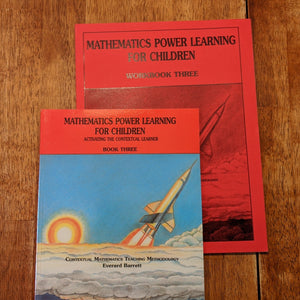Free Shipping on all U.S. Orders!# Level 3 Bundle (6th-8th)

Professor B

• \$57.00
Unit price per
Shipping calculated at checkout.

Contains Mathematics Power Learning Workbook 3, Mathematics Power Learning Book 3 (6th-8th). The Answer Key to Workbook Three is included free as a digital download with this purchase.

### Mathematics Power Learning for Children Level Three consists of two components:

1. Textbook
The textbook for use by the instructor has 165 pages. It contain clear, easy to follow instructions for presenting every lesson and concept. No teacher preparation is needed other than to glance over the lesson before you start. Even older children can teach younger children using this text.

2. Workbook
The workbook offers many unique practice exercises that make it possible for children to achieve the mastery which represents readiness for the next level. The 131-page workbook for Level 3 contains no endless pages of the same question. Purchase one workbook of each level per child. They are NOT reproducible.

## Scope and Sequence of Book 3

Covers: original and effective methods for thorough understanding and mastery of multiplication/division of fractions, the decimal and metric systems, operations on decimals, transforming among fractions/ decimals/percents and rounding off

• Multiplying fractions
• Dividing with fractions
• Structuring the computational experience with fractions and mixed numbers
• Comparing fractions
• Place value left and right of the decimal point
• Transforming fractions to equivalent decimals
• Place value relationships and the metric system
• Comparing decimals
• Adding, subtracting, multiplying, and dividing with decimals
• Transforming decimals to equivalent fractions
• Transforming percents into equivalent fractions
• Transforming fractions into equivalent percents
• Transforming percents into equivalent decimals
• Transforming decimals into equivalent percents
• Transforming among fractions, decimals, and percents
• Rounding off